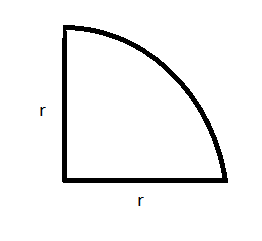QUESTION

# Calculate the perimeter and area of a quadrant of a circle whose radii arei) 98ii) 70iii) 42iv) 28

Hint: Perimeter can be defined as the total length of the boundary of a geometrical figure. Area can be defined as the space occupied by a flat shape or the surface of an object. So, using this definition we can easily solve our problem.

If a circle is drawn from the origin, then the quadrant of a circle can be defined as a portion of the circle which lies between the positive x-axis and positive y-axis.In general, the perimeter of the quadrant of circle $=\dfrac{\pi r}{2}+2r$.
The area of quadrant of a circle $=\dfrac{1}{4}\pi {{r}^{2}}$.
i) Perimeter of quadrant of a circle of radius 98 cm $=\dfrac{\pi (98)}{2}+2\times 98=350cm$
Area of quadrant of circle of radius 98 cm$=\dfrac{1}{4}\times \pi \times {{(98)}^{2}}=7546c{{m}^{2}}$
ii) Perimeter of quadrant of a circle of radius 70 cm $=\dfrac{\pi (70)}{2}+2\times 70=250cm$
Area of quadrant of circle of radius 70 cm$=\dfrac{1}{4}\times \pi \times {{(70)}^{2}}=3850c{{m}^{2}}$
iii) Perimeter of quadrant of a circle of radius 42 cm $=\dfrac{\pi (42)}{2}+2\times 42=150cm$
Area of quadrant of circle of radius 42 cm $=\dfrac{1}{4}\times \pi \times {{(42)}^{2}}=1386c{{m}^{2}}$
iv) Perimeter of quadrant of a circle of radius 28 cm $=\dfrac{\pi (28)}{2}+2\times 28=100cm$
Area of quadrant of circle of radius 28 cm $=\dfrac{1}{4}\times \pi \times {{(28)}^{2}}=616c{{m}^{2}}$

Note: The key step for solving this problem is the knowledge of area and perimeter of a geometrical figure. In this case, the given figure is a quadrant of a circle. So, on constructing the figure we obtain the area and parameter in generalized form. After putting values in the formula, we get the desired result.